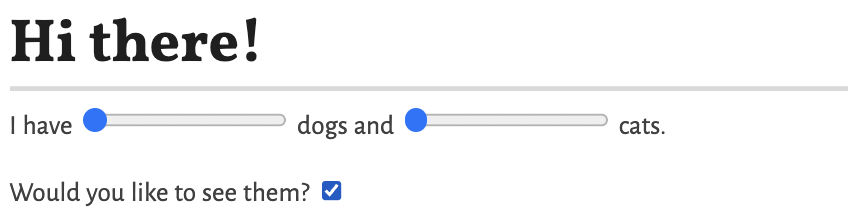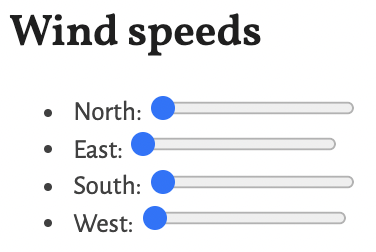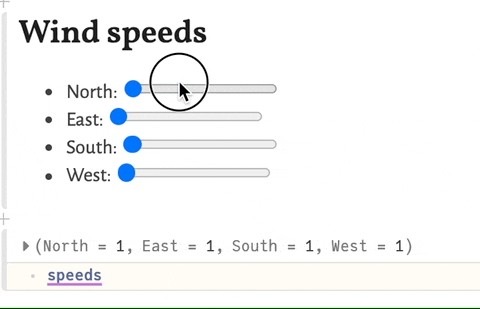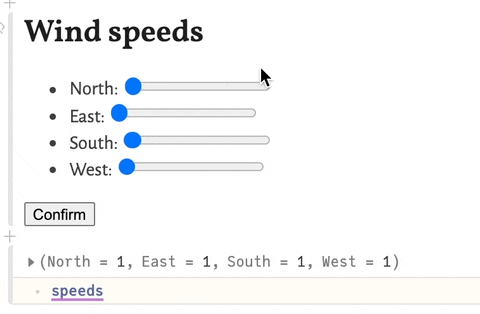PlutoUI.jl

# PlutoUI.jl# Essentials

## Number inputs

``````Slider
NumberField
Scrubbable
# RangeSlider``````

## String inputs

A text input (`<input type="text">`) - the user can type text, the text is returned as `String` via `@bind`.

If `dims` is a tuple `(cols::Integer, row::Integer)`, a `<textarea>` will be shown, with the given dimensions

Use `default` to set the initial value.

Examples

`@bind poem TextField()`

`@bind poem TextField((30,5); default="Hello JuliaCon!")`

A password input (`<input type="password">`) - the user can type text, the text is returned as `String` via `@bind`.

This does not provide any special security measures, it just renders black dots (•••) instead of the typed characters.

Use `default` to set the initial value.

Examples

`@bind secret_poem PasswordField()`

`@bind secret_poem PasswordField(default="Te dansen omdat men leeft")`

A color input (`<input type="color">`) - the user can pick an RGB color, the color is returned as color hex `String` via `@bind`. The value is lowercase and starts with `#`.

Use `default` to set the initial value.

Examples

`@bind color ColorStringPicker()`

`@bind color ColorStringPicker(default="#aabbcc")`

`ColorPicker`

A date input (`<input type="date">`) - the user can pick a date, the date is returned as `Dates.DateTime` via `@bind`.

Use `default` to set the initial value.

Examples

`@bind best_day_of_my_live DateField()`

`@bind best_day_of_my_live DateField(default=today())`

A time input (`<input type="time">`) - the user can pick a time, the time is returned as `String` via `@bind` (e.g. `"15:45"`). Value is `""` until a time is picked.

Use `default` to set the initial value.

Examples

`@bind lunch_time TimeField()`

`@bind lunch_time TimeField(default=now())`

## Selecting

A group of radio buttons - the user can choose one of the `options`, an array of `String`s.

`options` can also be an array of pairs `key::String => value::Any`. The `key` is returned via `@bind`; the `value` is shown.

Examples

`@bind veg Radio(["potato", "carrot"])`

`@bind veg Radio(["potato" => "🥔", "carrot" => "🥕"])`

`@bind veg Radio(["potato" => "🥔", "carrot" => "🥕"], default="carrot")`

``````Select(options::Vector; [default])
# or with a custom display value:
Select(options::Vector{Pair{Any,String}}; [default::Any])``````

A dropdown menu - the user can choose an element of the `options` vector.

See `MultiSelect` for a version that allows multiple selected items.

Examples

``@bind veg Select(["potato", "carrot"])``
``````@bind f Select([sin, cos, tan, sqrt])

f(0.5)``````

You can also specify a display value by giving pairs `bound_value => display_value`:

``````@bind f Select([cos => "cosine function", sin => "sine function"])

f(0.5)``````
``````MultiSelect(options::Vector; [default], [size::Int])
# or with a custom display value:
MultiSelect(options::Vector{Pair{Any,String}}; [default], [size::Int])``````

A multi-selector - the user can choose one or more of the `options`.

See `Select` for a version that allows only one selected item.

Examples

``````@bind vegetables MultiSelect(["potato", "carrot"])

if "carrot" ∈ vegetables
"yum yum!"
end``````
``````@bind chosen_functions MultiSelect([sin, cos, tan, sqrt])

[f(0.5) for f in chosen_functions]``````

You can also specify a display value by giving pairs `bound_value => display_value`:

``````@bind chosen_functions MultiSelect([
cos => "cosine function",
sin => "sine function",
])

[f(0.5) for f in chosen_functions]``````

The `size` keyword argument may be used to specify how many rows should be visible at once.

``@bind letters MultiSelect(string.('a':'z'), size=20)``
``MultiCheckBox(options::Vector; [default::Vector], [orientation ∈ [:row, :column]], [select_all::Bool])``

A group of checkboxes - the user can choose which of the `options` to return. The value returned via `@bind` is a list containing the currently checked items.

See also: `MultiSelect`.

`options` can also be an array of pairs `key::Any => value::String`. The `key` is returned via `@bind`; the `value` is shown.

Keyword arguments

• `defaults` specifies which options should be checked initally.
• `orientation` specifies whether the options should be arranged in `:row`'s `:column`'s.
• `select_all` specifies whether or not to include a "Select All" checkbox.

Examples

``````@bind snacks MultiCheckBox(["🥕", "🐟", "🍌"]))

if "🥕" ∈ snacks
"Yum yum!"
end``````
``````@bind functions MultiCheckBox([sin, cos, tan])

[f(0.5) for f in functions]``````
``@bind snacks MultiCheckBox(["🥕" => "🐰", "🐟" => "🐱", "🍌" => "🐵"]; default=["🥕", "🍌"])``
``@bind animals MultiCheckBox(["🐰", "🐱" , "🐵", "🐘", "🦝", "🐿️" , "🐝",  "🐪"]; orientation=:column, select_all=true)``

## Clicky click

A checkbox to choose a Boolean value `true`/`false`.

Examples

`@bind programming_is_fun CheckBox()`

`@bind julia_is_fun CheckBox(default=true)`

`md"Would you like the thing? \$(@bind enable_thing CheckBox())"`

A button that sends back the same value every time that it is clicked.

You can use it to trigger reactive cells.

See also `CounterButton` to get the number of times the button was clicked.

Examples

In one cell:

``@bind go Button("Go!")``

and in a second cell:

``````begin
# reference the bound variable - clicking the button will run this cell
go

md"My favorite number is 0.35803664437216476!"
end``````

A button that sends back the number of times that it was clicked.

You can use it to trigger reactive cells.

Examples

In one cell:

``@bind go CounterButton("Go!")``

and in a second cell:

``````begin
# reference the bound variable - clicking the button will run this cell
go

md"My favorite number is 0.3534388479470003!"
end``````

A button that sends back the same value every time that it is clicked.

You can use it to trigger reactive cells.

See also `CounterButton` to get the number of times the button was clicked.

Examples

In one cell:

``@bind go Button("Go!")``

and in a second cell:

``````begin
# reference the bound variable - clicking the button will run this cell
go

md"My favorite number is 0.35803664437216476!"
end``````

# Display helpers

Keyword arguments:

`title` header to this element, defaults to "Table of Contents"

`indent` flag indicating whether to vertically align elements by hierarchy

`depth` value to limit the header elements, should be in range 1 to 6 (default = 3)

`aside` fix the element to right of page, defaults to true

Examples:

``````TableOfContents()

TableOfContents(title="Experiments 🔬")

Line break without creating a new paragraph. Useful inside the `md"` macro:

Example

``````md"""
Hello \$br world!
"""``````
``Resource(src::String, mime=mime_from_filename(src)[, html_attributes::Pair...])``

A container for a URL-addressed resource that displays correctly in rich IDEs.

Examples

``Resource("https://julialang.org/assets/infra/logo.svg")``
``Resource("https://interactive-examples.mdn.mozilla.net/media/examples/flower.webm", :width => 100)``
``````md"""
This is what a duck sounds like: \$(Resource("https://interactive-examples.mdn.mozilla.net/media/examples/t-rex-roar.mp3"))
md"""``````

Create a `Resource` for a local file (a base64 encoded data URL is generated).

WARNING

`LocalResource` will not work when you share the script/notebook with someone else, unless they have those resources at exactly the same location on their file system.

Recommended alternatives (images)

1. Go to imgur.com and drag&drop the image to the page. Right click on the image, and select "Copy image location". You can now use the image like so: `PlutoUI.Resource("https://i.imgur.com/SAzsMMA.jpg")`.
2. If your notebook is part of a git repository, place the image in the repository and use a relative path: `PlutoUI.LocalResource("../images/cat.jpg")`.

Examples

``LocalResource("./cat.jpg")``
``LocalResource("/home/fons/Videos/nijmegen.mp4", :width => 200)``
``````md"""
This is what a duck sounds like: \$(LocalResource("../data/hannes.mp3"))
md"""``````

``Print(xs...)``

The text that would be printed when calling `print(xs...)`. Use `string(xs...)` if you want to use the result as a `String`.

See also: `Dump` and `with_terminal`.

``Dump(x; maxdepth=8)``

Every part of the representation of a value. The depth of the output is truncated at maxdepth.

This is a variant of `Base.dump` that returns the representation directly, instead of printing it to stdout.

See also: `Print` and `with_terminal`.

``with_terminal(f::Function; color::Bool=true, show_value::Bool=true)``

Run the function, and capture all messages to `stdout`. The result will be a small terminal displaying the captured text.

This allows you to to see the messages from `println`, `dump`, `Pkg.status`, etc.

Example:

``````with_terminal() do
x=1+1
println(x)
@warn "Oopsie!"
end ``````
``````with_terminal(show_value=false) do
@time x=sum(1:100000)
end ``````
``with_terminal(dump, [1,2,[3,4]])``

``````Show
WithIOContext
# as_html
# as_mime
# as_png
# as_svg
# as_text``````

# Special inputs

``````FilePicker
# Clock``````

# High-level components

``PlutoUI.combine(render_function::Function)``

Combine multiple input elements into one. The combined values are sent to `@bind` as a single tuple.

`render_function` is a function that you write yourself, take a look at the examples below.

Examples

🐶 & 🐱

We use the `do` syntax to write our `render_function`. The `Child` function is wrapped around each input, to let `combine` know which values to combine.

``````@bind values PlutoUI.combine() do Child
md"""
# Hi there!

I have \$(
Child(Slider(1:10))
) dogs and \$(
Child(Slider(5:100))
) cats.

Would you like to see them? \$(Child(CheckBox(true)))
"""
end

values == (1, 5, true) # (initially)``````

The output looks like:🎏

The `combine` function is most useful when you want to generate your input elements dynamically. This example uses HypertextLiteral.jl for the `@htl` macro:

``````function wind_speeds(directions)
PlutoUI.combine() do Child
@htl("""
<h6>Wind speeds</h6>
<ul>
\$([
@htl("<li>\$(name): \$(Child(Slider(1:100)))</li>")
for name in directions
])
</ul>
""")
end
end

@bind speeds wind_speeds(["North", "East", "South", "West"])

speeds == (1, 1, 1, 1) # (initially)

# after moving the sliders:
speeds == (100, 36, 73, 60)``````

The output looks like:Named variant

In the last example, we used `Child` to wrap around contained inputs:

``Child(Slider(1:100))``

We can also use the named variant, which looks like:

``Child("East", Slider(1:100))``

When you use the named variant for all children, the bound value will be `NamedTuple`, instead of a `Tuple`.

Let's rewrite our example to use the named variant:

``````function wind_speeds(directions)
PlutoUI.combine() do Child
@htl("""
<h6>Wind speeds</h6>
<ul>
\$([
@htl("<li>\$(name): \$(Child(name, Slider(1:100)))</li>")
for name in directions
])
</ul>
""")
end
end

@bind speeds wind_speeds(["North", "East", "South", "West"])

speeds == (North=1, East=1, South=1, West=1) # (initially)

# after moving the sliders:
speeds == (North=100, East=36, South=73, West=60)

md"The Eastern wind speed is \$(speeds.East)."``````

The output looks like:Why?

You can make a new widget!

You can use `combine` to create a brand new widget yourself, by combining existing ones!

In the example above, we created a new widget called `wind_speeds`. You could put this function in a package (e.g. `WeatherUI.jl`) and people could use it like so:

``````import WeatherUI: wind_speeds

@bind speeds wind_speeds(["North", "East"])``````

In other words: you can use `combine` to create application-specific UI elements, and you can put those in your package!

Difference with repeated `@bind`

The standard way to combine multiple inputs into one output is to use `@bind` multiple times. Our initial example could more easily be written as:

``````md"""
# Hi there!

I have \$(@bind num_dogs Slider(1:10)) dogs and \$(@bind num_cats Slider(5:10)) cats.

Would you like to see them? \$(@bind want_to_see CheckBox(true))
"""``````

The `combine` function is useful when you are generating inputs dynamically, like in our second example. This is useful when:

• The number of parameters is very large, and you don't want to write `@bind parameter1 ...`, `@bind parameter2 ...`, etc.
• The number of parameters is dynamic! For example, you can load in a table in one cell, and then use `combine` in another cell to select which rows you want to use.
``confirm(element::Any)``

Normally, when you move a `Slider` or type in a `TextField`, all intermediate values are sent back to `@bind`.

By wrapping an input element in `confirm`, you get a button to manually control when the value is sent, intermediate updates are hidden from Pluto.

One case where this is useful is a notebook that does a long computation based on a `@bind` input.

Examples

``````@bind x confirm(Slider(1:100))

x == 1 # (initially)``````

Now, when you move the slider, nothing happens, until you click the `"Confirm"` button, and `x` is set to the new slider value.

The result looks like:You can combine this with `PlutoUI.combine`!

``````@bind speeds confirm(
combine() do Child
@htl("""
<h3>Wind speeds</h3>
<ul>
\$([
@htl("<li>\$(name): \$(Child(name, Slider(1:100)))")
for name in ["North", "East", "South", "West"]
])
</ul>
""")
end
)``````

The result looks like: